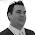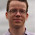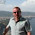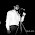All my life I've done mental arithmetic the 'wrong way': I've calculated from left-to-right, instead of right-to-left. So when I do something like 24 + 35 I'll see the 50 first and then the 9. This even applies when there's a carry and I'll do something like 36 + 56 as 80 + 12. I do the same thing for multiplication as well.

Turns out I'm not so weird after all (well, apart from the finding doing mental arithmetic fun bit). I've been reading Secrets of Mental Math: The Mathemagician's Guide to Lightning Calculation and Amazing Mental Math Tricks and the author, Michael Shermer, is just like me: he works from left-to-right.

He, like me, has found this to be a good system because it lets you discard digits early and not hold some enormous calculation in your head. For example, in the calculation 124 + 353 you can immediately say "four hundred" before doing the rest of the sum. This seems to free up headspace (at least for me) and let's me do the rest of the calculation. I'd do it like this: 124 + 353 = 400 + 24 + 53 = 400 + 70 + 4 + 3 = 477.

The books is filled with tricks for doing all sorts of mental calculations, including a nice section about estimation (I've always been an estimator) and working out things like tips and sales taxes. But the most fun part to me was a trick to let you do two-digit squares in your head really, really fast.

Quick, what's 272. Of course, the brute force way to do that is to calculate 27 x 27 which is a bit of a pain because it involves doing something like 27 x 20 + 27 x 7 = 540 + 189 = 729. But there's a much faster way.

Observe that 272 = 30 x 24 + 32. Since you probably know that 32 = 9 this means you have to calculate 30 x 24 + 9 which is relatively easy because the multiplication involves a multiple of ten which means it's really 3 x 24 and then add a zero.

So the rule is that if you want to square number X you first round it to the nearest multiple of 10, called that X + r, and then calculate X - r (i.e. round the same amount in the opposite direction). You calculate (X + r) x (X - r) and add back the square of the amount you rounded by, r2, which will be 1, 4, 9, 16 or 25.

This works because ( X + r ) x ( X - r ) + r2 = X2 - rX + rX - r2 + r2 = X2.

Example: 672 is 70 x 64 + 32 which is fairly easy to do in your head. And naturally the same trick works no matter how many digits you have, it's just that the multiplication gets harder.

The trick is especially impressive/easy with numbers near 100 because the multiplication becomes dead easy. For example, 962 is 100 x 92 + 42 which you (or at least I) can almost instantly see is 9216.Andrew said…
So easy! I just stuck this one in my memory and practised on paper for a bit. Will be exceptionally handy in the future.

Have also put that book on my 'To read' list.ramanr said…
you have something similar to this in Vedic MathematicsUnknown said…
Google Trachtenberg and Mathematics, something similar.Rakesh Pai said…
I've used a similar technique for squaring two digit numbers really easily.

A two digit number can be represented as 10x + y, where x and y are the first and second digits. Squaring 10x + y gives us 100x(2) + 2*10xy + y(2). This is very simple now to compute. The result of y(2) will always be the last digit since the first two numbers have been offset by their multiplications by 10. The second digit will always be 2xy since it's offset by 10. The first digit will be x(2) since it's offset by 100.

Example: say 13 squared. 3 is the last digit, so the last digit of the square will be 9. 2x1x3 = 6, which will be digit before 9, giving us 69 so far. 1 squared is 1, and the answer is 169.

Another example: 57 squared. 7 squared is 49, so 9 is the last digit, and we carry 4 over. 2x5x7 = 70. We add the carried over 4 to get 74. So far we have 49 then, with 7 carried over. 5 squared is 25. +7 to give 32. The answer is 3249.Rakesh Pai said…
Oh, and in the 10x+y example, if you want to deal with a special case where the last digit is 5 (y=5), the squaring becomes simpler still.

100x(2) + 2*10x*5 + 5(2)
= 100x(2) + 100x + 25
= 100x(x + 1) + 25

So, the last digit is always 25 since there's an offset of 100 contributed by the first digit. The first digit is x(x+1), which is very simple to compute.

For example, 25 squared. 2x3 = 6. The answer is 625.
Another example: 85 squared. 8x9 = 72. Answer = 7225.Jason said…
Any two digit number ending in 5 is easy.

The answer always ends in 25, and the first part is handled by taking the first number and multiplying it by the number higher.

So, 95x95 is 9025. 9 * 10 = 90 and put 25 on the end.Anonymous said…
Oddly enough, I often do mental arithmetic the wrong way round as well. The cognitive scientists reckon that working memory (like RAM) is the limiting factor on your performance, so perhaps it makes sense to minimise how much of it you're using. Not that I intentionally set out to achieve that.martijn said…
Ever heard of Arthur Benjamin? He is a mathemagician, which probably describes best what he does: magic tricks using maths. Lots of impressive calculations by head, but most of them are based on tricks like this.

My favourite one -- actually, the only one I remember from the presentation I saw 8 years ago -- is how to remember really larger numbers: phone numbers, digits of pi, etc. Assign each digit to a vowel sound (e.g. 0 = long o, 1 = short a etc.) and then make sentences of which the vowel sounds match the digits of the number you want to memorize. Never needed to use that in practise though.Brute Force said…
I find that my method is simpler for me when I square 2-digit numbers. Not sure it's like that for everyone.

When I square 2-digit numbers I always round down to the nearest 10. Then, add the double of the criss-cross product. And, add the final square.

Examples:
27*27
Round down to 20*20 = 400.
Criss-cross is 20*7 = 140.
Double it = 280. Now, we have 400+280 = 680, and we just need to add the final square = 7*7 = 49.

For me, addition is always easiest, and doubling something is also very natural.

96*96 = 8100 + Double(540) + 36 = 8100 + 1080 = 9180. Add 36 = 9216.

It probably depends on the person which method is simpler. But, this is the way I've always done it.bigfun said…
Hmm, now i feel quite proud because in Poland such things are taught in primary school :)Charles Wood said…
I did your 27 squared differently.

I noted that 27 = 3x9 so I squared 3 and 9 to get 9 and 81. Multiplying together gave 9 x 80 = 720 and 9 x 1 = 9 result 729. Perhaps 5 seconds thought.

Prime factoring usually brings the numbers back into the times tables range - barring larger primes being present.Catch light said…
I'm 41 and I have always done math in my head by breaking it down like this. Unfortunately, I didn't do well back in high school because they didn't seem to care about the answer if I didn't use their methods =(Unknown said…
You can use this info in a trick for approximating square roots. I've never had to use this in a real world situation, but amongst drunk nerds, it has gained me a few bucks in bets.

So, say for example you want to approximate the square root of 750. From your trick above, you could find that the 750 is between 27^2 and 28^2, or 729 and 784. So you know the square root of 750 is at least 27.x.

Notice that the difference between 28^2-27^2 = (28+27)-(28-27) = 28+27 = 55. Also that the difference between are target and the lower number is 750-729 = 21.

So if we fit a line between our two square numbers and compare the slope between our two squares and our lower square to our target, we get 55/1 = 21/x. Solve for x, you get 21/55. That's slightly more than 20/55, which is 4/11, which is .36. That 1/55 slightly less than .02, so we'd approximate our x to be .38. So then our square root approximation is 27.38.

Checking myself, the square root of 750 = 27.3861, so I got close. This approximation always falls a tad short, especially the closer you get to 0. In fact, it's really a bad approximation anything less than 100, but it's close. It's because the curve of the parabola y=x^2 will actually go under your line you have created, and that difference gets worse the smaller x is.

Another example, 600 is between 24^2 and 25^2 or between 576 and 625. So square root of 600 is 24.x. That x is (600-576)/(24+25 or 625-576) = 24/49. 24.5/49 is .5, so 24/49 is .5-.5/49 = .5-1/98 which is approx. .5-.01=.49. So my guess is 24.49. Actual is 24.4948.Saw this post via Lifehacker. Ordered the book off Amazon, nice!Mohan Arun said…
There are even more math tricks and shortcuts for mental math in a book called "Vedic Mathematics" (published by Motilal Banarsidass from India)Unknown said…
This is actually quite natural – a lot of us tend to think left to right, instead of the traditional right to left method. I completely agree with the post – this approach makes calculations a lot simpler and faster, and in turn, also sharpens mental math skills. But then, isn’t it built in for some people, and not for others? Anyway, that’s a separate discussion in itself!

I love the (x-r)*(x+r)+r^2 method… this actually makes squaring in your head so much quicker, and this is one of those tricks that not many people know of. Thanks!

Dan Sherman
http://www.tenmarks.comJerbear said…
WHAT! WHAT! WHAT! This makes no sense whatsoever to me.....27 rounded to 30 easy....but after that my brain fried.....this is as clear as mud!!!Jerbear said…
WHAT! WHAT! WHAT! This makes no sense whatsoever to me.....27 rounded to 30 easy....but after that my brain fried.....this is as clear as mud!!!Jerbear said…
WHAT! WHAT! WHAT! This makes no sense whatsoever to me.....27 rounded to 30 easy....but after that my brain fried.....this is as clear as mud!!!SUnny said…
http://pythonnotesbyajay.blogspot.in/2015/03/patterns-finding-squares-in-hard-way.html

I've observed this pattern.Unknown said…
Glad I dont have trouble squaring 2 or 3 digit numbers in my headUnknown said…
How to do 26th squareUnknown said…
How to do 26th square in this waykethees kethees: 26^2 = 30 * 22 + 4^2 = 660 + 16 = 676.onnet said…
There is another easy way to find square quick & Easy by chinese method
https://youtu.be/zFTnPvS4sioonnet said…
There is another easy way to find square quick & Easy by chinese method
https://youtu.be/zFTnPvS4sio

### Your last name contains invalid characters

My last name is "Graham-Cumming". But here's a typical form response when I enter it: Does the web site have any idea how rude it is to claim that my last name contains invalid characters? Clearly not. What they actually meant is: our web site will not accept that hyphen in your last name. But do they say that? No, of course not. They decide to shove in my face the claim that there's something wrong with my name. There's nothing wrong with my name, just as there's nothing wrong with someone whose first name is Jean-Marie, or someone whose last name is O'Reilly. What is wrong is that way this is being handled. If the system can't cope with non-letters and spaces it needs to say that. How about the following error message: Our system is unable to process last names that contain non-letters, please replace them with spaces. Don't blame me for having a last name that your system doesn't like, whose fault is that? Saying "Your

### How to write a successful blog post

First, a quick clarification of 'successful'. In this instance, I mean a blog post that receives a large number of page views. For my, little blog the most successful post ever got almost 57,000 page views. Not a lot by some other standards, but I was pretty happy about it. Looking at the top 10 blog posts (by page views) on my site, I've tried to distill some wisdom about what made them successful. Your blog posting mileage may vary. 1. Avoid using the passive voice The Microsoft Word grammar checker has probably been telling you this for years, but the passive voice excludes the people involved in your blog post. And that includes you, the author, and the reader. By using personal pronouns like I, you and we, you will include the reader in your blog post. When I first started this blog I avoid using "I" because I thought I was being narcissistic. But we all like to read about other people, people help anchor a story in reality. Without people your bl

### The Elevator Button Problem

User interface design is hard. It's hard because people perceive apparently simple things very differently. For example, take a look at this interface to an elevator: From flickr Now imagine the following situation. You are on the third floor of this building and you wish to go to the tenth. The elevator is on the fifth floor and there's an indicator that tells you where it is. Which button do you press? Most people probably say: "press up" since they want to go up. Not long ago I watched someone do the opposite and questioned them about their behavior. They said: "well the elevator is on the fifth floor and I am on the third, so I want it to come down to me". Much can be learnt about the design of user interfaces by considering this, apparently, simple interface. If you think about the elevator button problem you'll find that something so simple has hidden depths. How do people learn about elevator calling? What's the right amount of